There are times when I have an Excel worksheet full of formulas and I want to hard code the results and Excel remove formula completely.

This is very easy to do! Keep reading to know more.

Removing formulas and keeping the data in Excel may be necessary because of the following reasons:

• You don’t require the value to be linked to any other cells and want to display just the value.
• You may have confidential information inside the formula that you don’t want to disclose and it is best to Excel remove formula.

Whatever be the reason, sometimes it becomes extremely essential to remove formulas from your Excel workbook and show just the values.

Here is our sample worksheet which has the following formulas in Column E: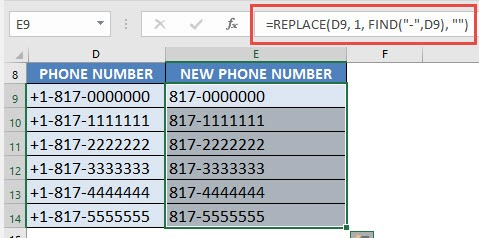Instead of showing the formula =REPLACE(D9, 1, FIND(“-“,D9), “”) in the address bar, you want the value i.e. the new phone number to be displayed.

This can easily be done in Excel using either of the two methods:

### Remove Formula using Right Click

Watch it on YouTube and give it a thumbs-up!STEP 1: Select all the cells that have formulas:STEP 2:Right click and select Copy: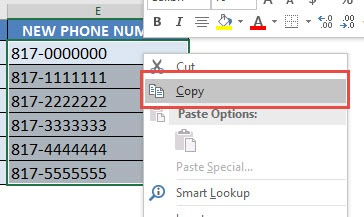STEP 3: Right click again and select Paste Values: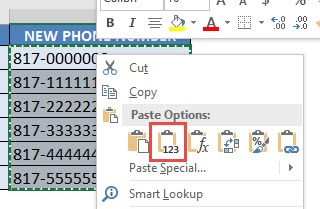Now you will see that the values are only retained and the formulas are now gone!

### Remove Formula using the Home Tab

STEP 1: Select all the cells that have formulas:STEP 2: Go to the Home tab in the Clipboard group, click the arrow below Paste and then click Paste Values.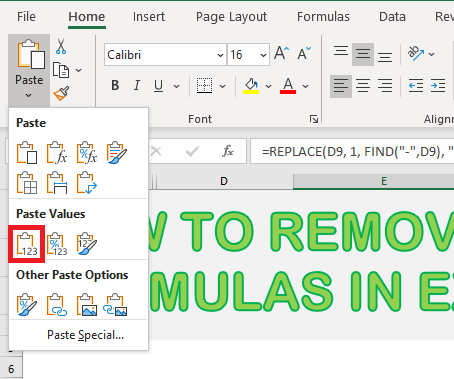Now, you will see that the cells don’t have formulas anymore. It has been replaced by values.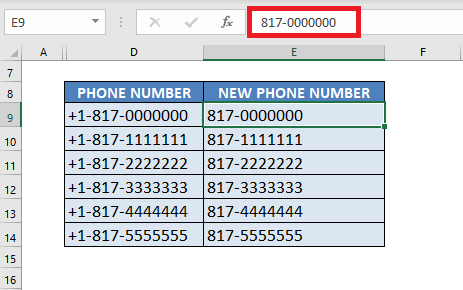Lastly, you can even use keyboard shortcuts to convert formulas to value.

### Find & Remove Formula using Keyboard Shortcuts

In the above scenarios, you already knew which cells contain formulas.

Let’s go to an example where you have to first find the cells containing formulas and then learn how to remove formulas in Excel using keyboard shortcuts.

In the data below, the sales price for some product have been calculated using formulas and some are constant values.

You have to remove the formulas from your Excel Worksheet. Follow the steps below to learn how to remove formula in Excel shortcut:

STEP 1: Press Ctrl + G to open the Go To dialog box and then select Special.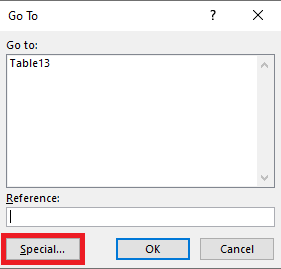STEP 2: Select Formulas for the list and click OK.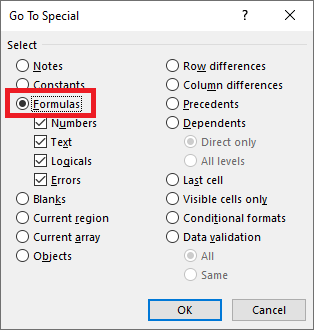All the cells containing formulas will be selected.

STEP 3: Highlight these cells with color.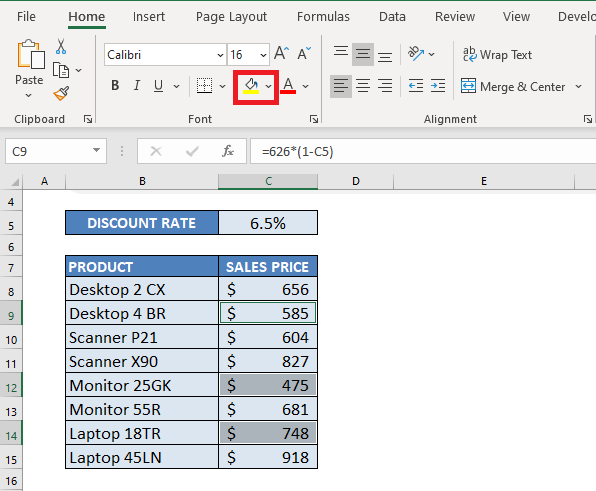The cells will be highlighted in yellow!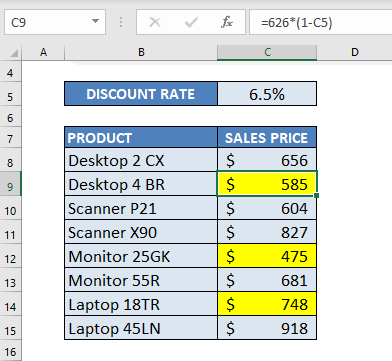STEP 4: Press Ctrl + C to copy the cells.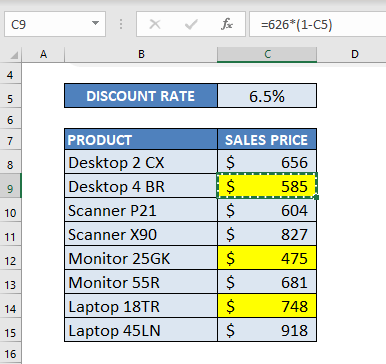STEP 5: Press Alt+E+S to open the Paste Special option dialog box.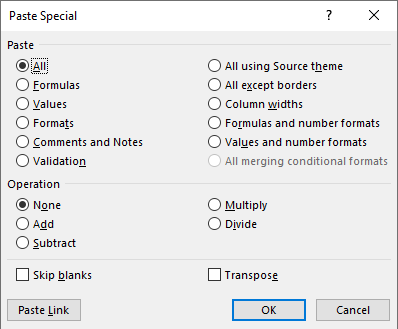STEP 6: Press V to select values and click OK.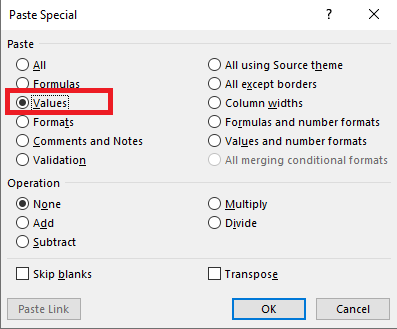The formula has now been replaced with value.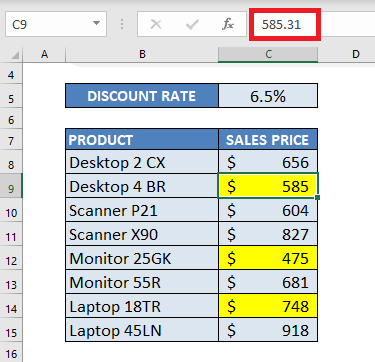STEP 7: Repeat the same steps for other highlighted cells.

### Conclusion

There are 3 ways in which you can Excel remove formula using either right-click, home tab, or keyboard shortcuts. This will help you to remove the formula without deleting the data.

You can easily reduce the size of your file or hide the formula from recipients!